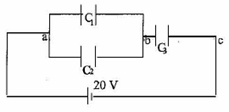# Problem: Three capacitors C1 = 4μF, C2 =8μF, and C3 = 6μF are connected across a 20 V battery as shown. a) Find the voltage drop across C1. b) Find the voltage drop across C2. c) Find the voltage drop across C3. d) Find the charge on across C2. e) Find the charge on across C3.

###### FREE Expert Solution
84% (204 ratings)
###### Problem Details

Three capacitors C1 = 4μF, C2 =8μF, and C3 = 6μF are connected across a 20 V battery as shown.

a) Find the voltage drop across C1.

b) Find the voltage drop across C2.

c) Find the voltage drop across C3.

d) Find the charge on across C2.

e) Find the charge on across C3.